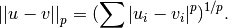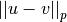# scipy.spatial.distance.minkowski¶

scipy.spatial.distance.minkowski(u, v, p)[source]

Computes the Minkowski distance between two 1-D arrays.

The Minkowski distance between 1-D arrays u and v, is defined asParameters : u : (N,) array_like Input array. v : (N,) array_like Input array. p : int The order of the norm of the difference. d : double The Minkowski distance between vectors u and v.

#### Previous topic

scipy.spatial.distance.matching

#### Next topic

scipy.spatial.distance.rogerstanimoto